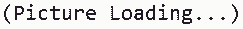You've successfully subscribed to The Finbox Blog
Welcome back! You've successfully signed in.# WACC: Weighted Average Cost of Capital Explained

### What is WACC and What Does it Tell Us?

By definition, the weighted average cost of capital (WACC) is the average after-tax cost of a company's various capital sources. These include preferred stock, common stock, bonds, and long-term debt. So, as the name implies, WACC is the average rate that a company pays to finance its assets.

Since almost every business needs to raise capital to grow, WACC is one of the most important financial indicators. It represents the expense of raising money—so the higher it is, the lower a company's net profit. For instance, a WACC of 10% means that a business will have to pay its investors an average of \$0.10 in return for every \$1 in extra funding.### How To Calculate WACC: Formula Explained

So, now that you’ve got an idea of what it is, let's see how to calculate WACC with the right formula. WACC is calculated by multiplying each capital source's cost by the corresponding weight and by adding the products together to get the weighted average cost of capital.

Obviously, the more complex the company’s capital structure is, the more complex the WACC calculation. The main capital sources of most publicly traded companies are usually debt and common stocks. Here's the WACC formula:

WACC = E/TC*Re + D/TC*Rd*(1 – Tax Rate)
• E = Market value of the firm’s equity
• TC (Total Capital) = Total market value of the firm’s financing (Equity + Debt)
• Re = Cost of equity
• D = Market value of the firm’s debt
• Rd = Cost of debt

### WACC Example Calculation

As an example calculation, we can use the formula above to calculate Apple's (NASDSAQGS:AAPL) weighted average cost of capital. Using the Finbox data explorer, we can find the values of the metrics required to apply the formula:

We can now perform a WACC example calculation for Apple:

• Apple's Equity (% Of Total Capital) = Apple's Market Cap/Total Capital = \$2,064.4 billion / \$2186.6 billion = 94.4%
• Apple's Cost Of Equity = Risk free rate + Apple's βeta*Equity Premium = 2.5%+0.97*6%=8.32%
• Apple's Debt (% Of Total Capital) = Apple's Debt/Total Capital = \$122.2 billion / \$2186.6 billion = 5.6%
Apple's WACC = Apple's Equity (% Of Total Capital) * Apple's Cost Of Equity + Apple's Debt (% Of Total Capital) * Apple's Cost Of Debt
= 94.4% * 8.32% + 5.6% * 4.5%
= 8.1%

### WACC Calculator - Free Excel Template

You can calculate CAPM and WACC for every single company in the world using this template. You just have to enter the required data of the company whose ratio you want to calculate, and the model will do everything automatically.

You can use the Finbox Data Explorer to get all the data you need for 100,000+ companies worldwide. Here's a preview of Finbox's WACC Calculator:### WACC: Sector Benchmarks

As of October 16, 2020, the sectors with the highest WACC are healthcare, energy, and materials. Utilities, financials, and real estate are the sectors with the lowest WACC.### What is a Good WACC?

If you try searching "what is a good WACC" on Google, you will find a wide range of answers. One way to easily determine a good WACC is to look at the sector average. As you can see in the picture above, the weighted average cost of capital varies considerably from one sector to another, ranging from more than 10% for healthcare to 7% for utilities.

That's because investors and lenders consider utility stocks less risky than the market, so they require a lower rate of return to finance those companies with both equity and debt.

As a rule of thumb, a good WACC is one that is in line with the sector average. When investors and lenders require a higher rate of return to finance a company it may indicate that they consider it riskier than the sector.

Tags